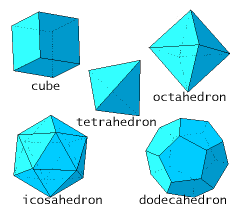Logic puzzles, riddles, math puzzles and brainteasers - pzzls.com

Vandaag is het 26 September 2023

Sort - Most difficult on top
Page: << 1 2 3 4 5 >>

# Somewhere on a sphere - math puzzle

Difficulty:Rating: 2.7/5.0

What is the mean distance between two randomly choosen points within a sphere of radius 1?

# Platonic solids - math puzzle

Difficulty:Rating: 2.8/5.0

 Show that there exist only five platonic solids (that are objects which are build out of the one sort of regular polygons, like the tetrahedron and the cube). Hint: use that for each solid in three dimensions it holds that the number of vertices + the number of faces is equal to the number of edges + 2.# Divisible from 1 to 9 - math puzzle

Difficulty:Rating: 3.6/5.0